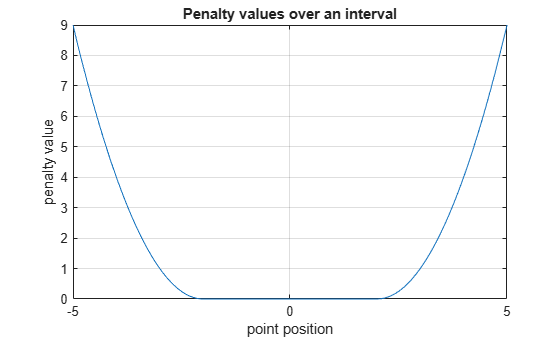# exteriorPenalty

Exterior penalty value for a point with respect to a bounded region

## Syntax

``p = exteriorPenalty(x,xmin,xmax,method)``

## Description

example

````p = exteriorPenalty(x,xmin,xmax,method)` uses the specified `method` to calculate the nonnegative (exterior) penalty vector `p` for the point `x` with respect to the region bounded by `xmin` and `xmax`. `p` has the same dimension as `x`.```

## Examples

collapse all

This example shows how to use the `exteriorPenalty` function to calculate the exterior penalty for a given point, with respect to a bounded region.

Calculate the penalty value for the point `0.1` within the interval [`-2`,`2`], using the `step` method.

`exteriorPenalty(0.1,-2,2,'step')`
```ans = 0 ```

Calculate the penalty value for the point `4` outside the interval [`-2`,`2`], using the `step` method.

`exteriorPenalty(4,-2,2,'step')`
```ans = 1 ```

Calculate the penalty value for the point `4` outside the interval [`-2`,`2`], using the `quadratic` method.

`exteriorPenalty(4,-2,2,'quadratic')`
```ans = 4 ```

Calculate the penalty value for the point [`-2`,`0`,`4`] with respect to the box defined by the intervals [`0`,`1`], [`-1`,`1`], and [`-2`,`2`] along the x, y, and z dimensions, respectively, using the `quadratic` method.

`exteriorPenalty([-2 0 4],[0 -1 -2],[1 1 2],'quadratic')`
```ans = 3×1 4 0 4 ```

Visualize Penalty Values for an Interval

Create a vector of 1001 equidistant points distributed between -`5` and `5`.

`x = -5:0.01:5;`

Calculate penalties for all the points in the vector, using the `quadratic` method.

`p = exteriorPenalty(x,-2,2,'quadratic');`

Plot the points and add grid, labels, and title.

```plot(x,p) grid xlabel("point position"); ylabel("penalty value"); title("Penalty values over an interval");```## Input Arguments

collapse all

Point for which the exterior penalty is calculated, specified as a numeric scalar, vector, or matrix.

Example: `[-0.1, 1.3]`

Lower bounds for `x`, specified as a numeric scalar, vector, or matrix. To use the same minimum value for all elements in `x`, specify `xmin` as a scalar.

Example: `-2`

Upper bounds for `x`, specified as a numeric scalar, vector, or matrix. To use the same maximum value for all elements in `x`, specify `xmax` as a scalar.

Example: `[5 10]`

Function used to calculate the penalty, specified either as `'step'` or `'quadratic'`. You can also use strings instead of character vectors.

Example: `"quadratic"`

## Output Arguments

collapse all

Penalty value, returned as a vector of nonnegative elements. With either of the two methods, each element `pi` is zero if the corresponding `xi` is within the region specified by `xmini` and `xmaxi`, and it is positive otherwise. Penalty functions are typically used to generate negative rewards when constraints are violated, such as in `generateRewardFunction`.